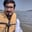Related Tags

python
pandas
column
communitycreator

# How to create lags and leads of a column in a pandas DataFrameArslan Bajwa

In Python, the pandas library includes built-in functionalities that allow you to perform different tasks with only a few lines of code. One of these functionalities is the creation of lags and leads of a column.

• lag shifts a column down by a certain number.
• lead shifts a column up by a certain number.

## Input

 ID 1 2 3 4 5 6

## Output

 ID Leads 1 Lags 1 1 2 NaN 2 3 1 3 4 2 4 5 3 5 6 4 6 NaN 5

## Method

In this method, we first initialize a pandas dataframe with a numpy array as input. Then we select a column and apply lead and lag by shifting that column up and down, respectively.

#importing pandas and numpy libraries
import pandas as pd
import numpy as np

#initializing pandas dataframe with numpy arrays
df = pd.DataFrame(np.random.randint(1, 100, 10).reshape(-1, 2), columns = list('ab'))

#applying leads and lags by shifting dataframe column up and down respectively
df['lag(a,1)'] = df['a'].shift(1)

df['lag(a,5)'] = df['a'].shift(5)

print(df)

RELATED TAGS

python
pandas
column
communitycreator

CONTRIBUTORArslan Bajwa
RELATED COURSES

View all Courses

Keep Exploring

Learn in-demand tech skills in half the time